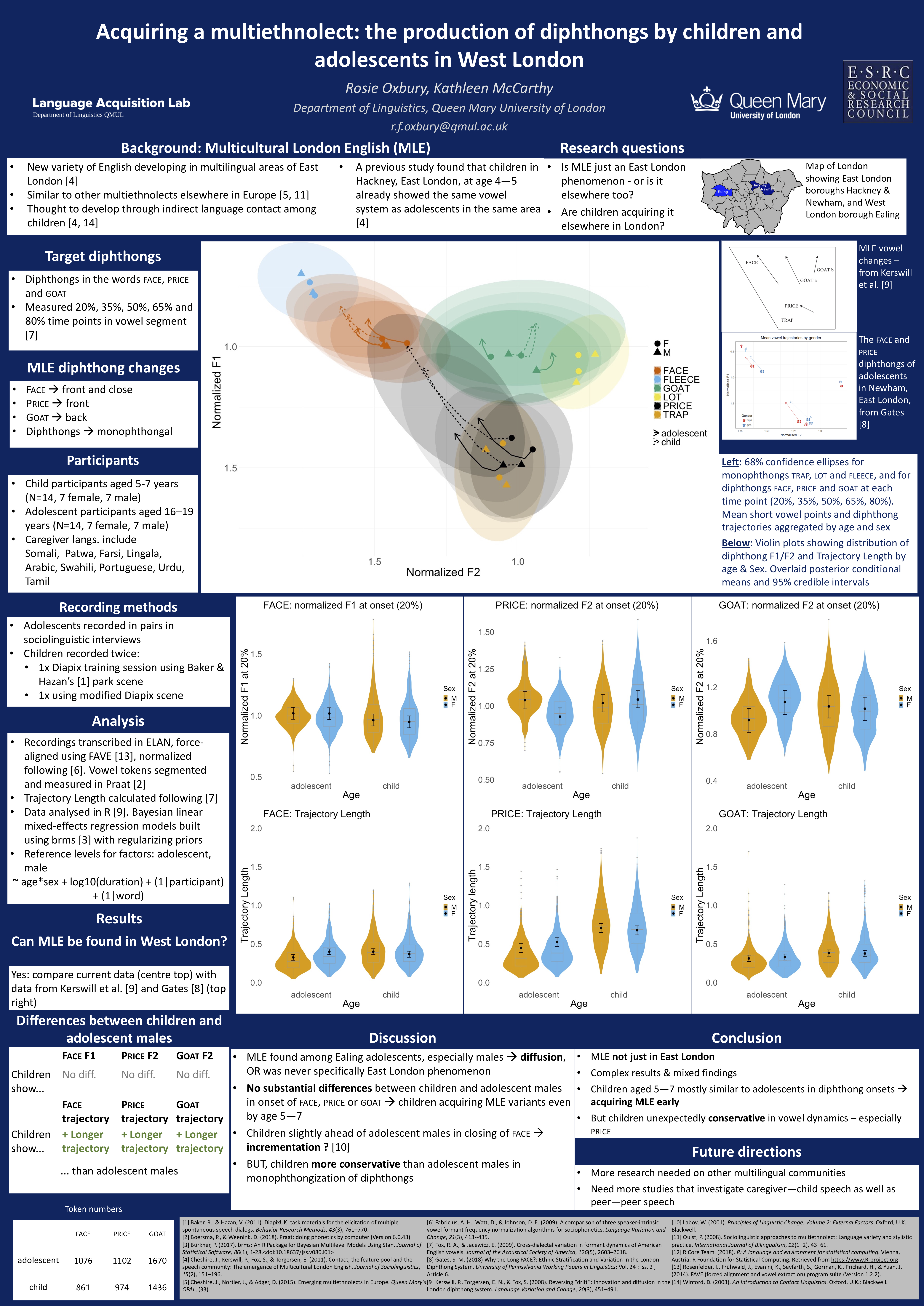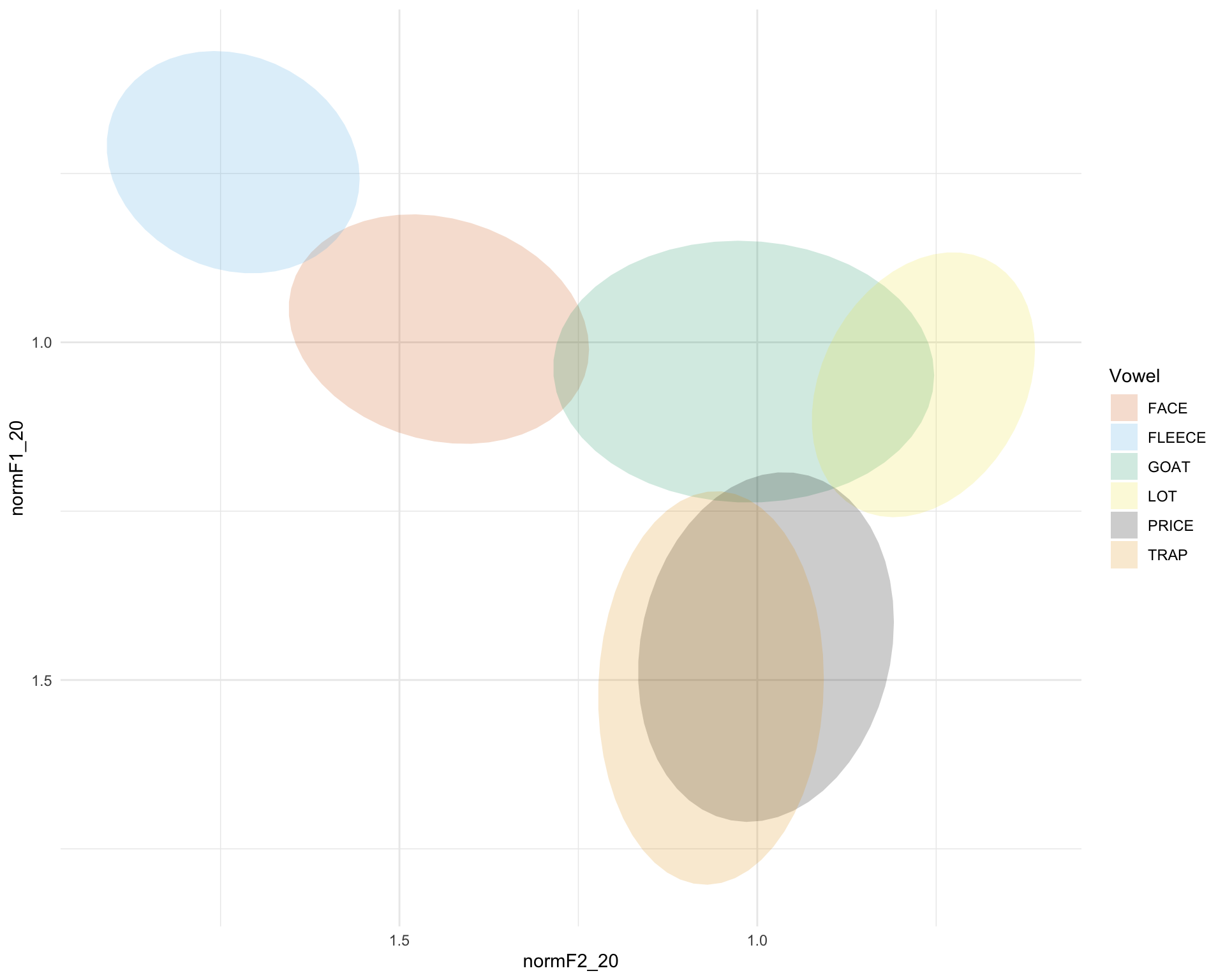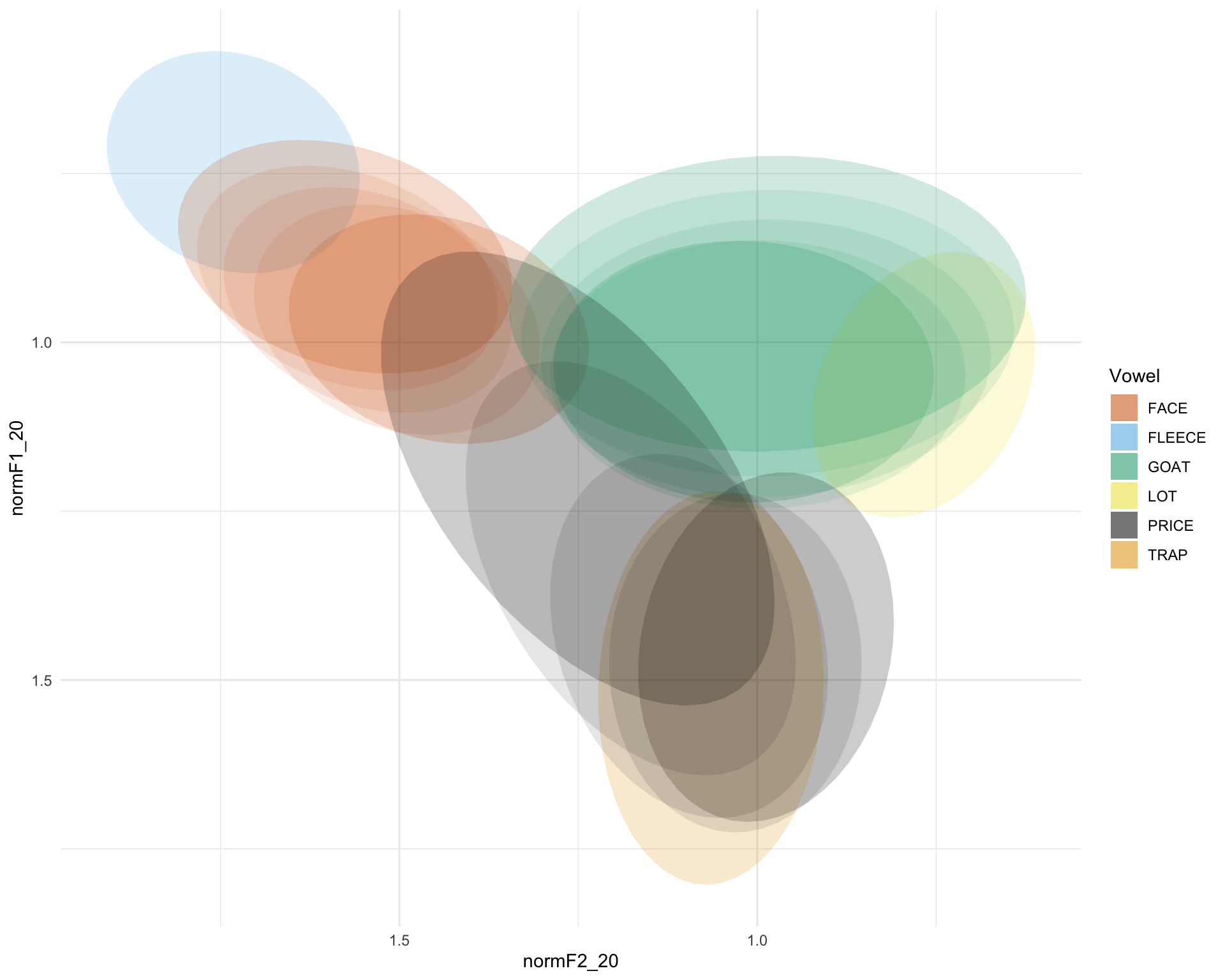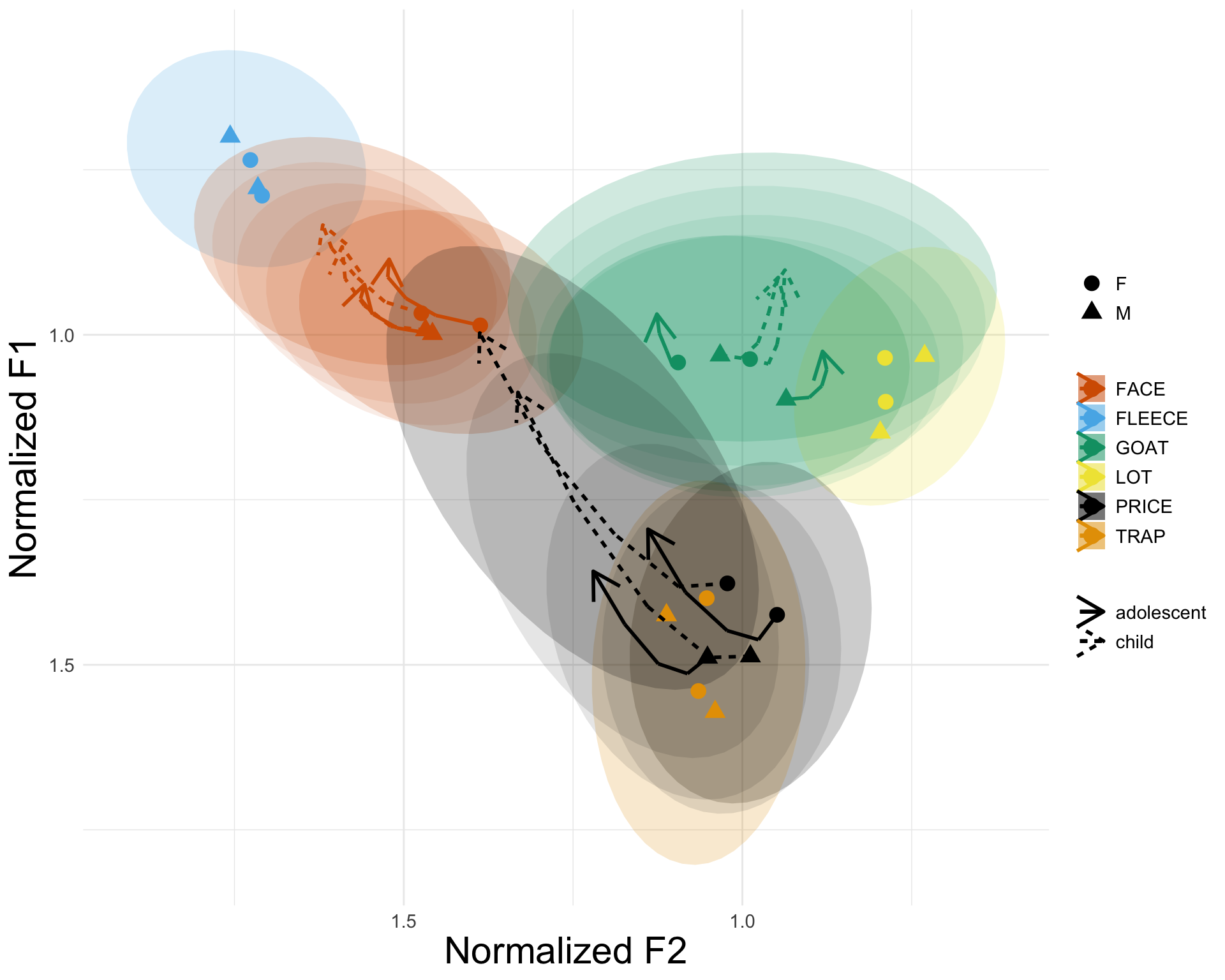# 1 Introduction

This notebook provides the code for the vowel plot that was shown on Oxbury & McCarthy’s ICPhS poster.# 2 Data cleaning & preparation

As a first step, of course, load tidyverse.

library(tidyverse)
## ── Attaching packages ───────────────────────────────── tidyverse 1.3.0 ──
## ✓ ggplot2 3.3.1     ✓ purrr   0.3.4
## ✓ tibble  3.0.1     ✓ dplyr   1.0.0
## ✓ tidyr   1.1.0     ✓ stringr 1.4.0
## ✓ readr   1.3.1     ✓ forcats 0.5.0
## ── Conflicts ──────────────────────────────────── tidyverse_conflicts() ──
## x dplyr::lag()    masks stats::lag()

data <- read_csv("icphs_data.csv")
## Parsed with column specification:
## cols(
##   .default = col_double(),
##   participant = col_character(),
##   sound_label = col_character(),
##   word = col_character(),
##   age = col_character(),
##   gender = col_character(),
##   rol.var = col_character(),
##   face.l = col_character(),
##   price.l = col_character()
## )
## See spec(...) for full column specifications.

Make a grouping variable.

data <- data %>% mutate(
group = ifelse(
gender=="M" & age=="child", "Ch_M", ifelse(
)
)
)
)

Check that it worked

table(data$group) ## ## Ad_F Ad_M Ch_F Ch_M ## 2466 1759 2048 2282 Convert sound_label to a factor data$sound_label <- as.factor(data$sound_label) Check levels levels(data$sound_label)
##   "dress"  "face"   "fleece" "foot"   "goat"   "goose"  "kit"    "lot"
##   "mouth"  "price"  "start"  "strut"  "trap"

But we don’t want all of these vowels. We will create a vector of the names of the vowels we are interested in – the diphthongs FACE, PRICE and GOAT, and the reference vowels FLEECE, LOT and TRAP – so that then we can subset these out.

vowels <- c("face",
"fleece",
"goat",
"lot",
"price",
"trap")

Now create the subset:

sub_data <- data %>% filter(sound_label %in% vowels)

Check that this worked:

table(sub_data$sound_label) ## ## dress face fleece foot goat goose kit lot mouth price start ## 0 1937 304 0 2076 0 0 296 0 3106 0 ## strut trap ## 0 293 Rename sound_label as Vowel – that is what it should be called! sub_data <- sub_data %>% rename( Vowel = sound_label ) And rename the levels of “Vowel” so that they are in capitals. First, check what the levels are at present: levels(sub_data$Vowel)
##   "dress"  "face"   "fleece" "foot"   "goat"   "goose"  "kit"    "lot"
##   "mouth"  "price"  "start"  "strut"  "trap"

And then rename these:

levels(sub_data\$Vowel) <- c("dress",
"FACE",
"FLEECE",
"foot",
"GOAT",
"goose",
"kit",
"LOT",
"mouth",
"PRICE",
"start",
"strut",
"TRAP")

# 3 Plotting

First of all, define a colourblind friendly palette:

cbbPalette <- c("#D55E00", "#56B4E9", "#009E73", "#F0E442", "#000000", "#E69F00")

Create an initial plot of just ellipses:

a <-  sub_data %>% ggplot(aes(x=normF2_20,
y=normF1_20,
group = Vowel,
color = Vowel,
fill = Vowel,
shape = gender,
linetype = age)) +
scale_x_reverse() +
scale_y_reverse() +
stat_ellipse(geom = "polygon",
alpha = 0.2,
linetype = 0,
level=0.67) +
theme_minimal() +
scale_fill_manual(values=cbbPalette) +
scale_colour_manual(values=cbbPalette)
aTry adding ellipses for diphthong finishing points

just.diph <- sub_data %>% filter(Vowel == "FACE" | Vowel == "PRICE" | Vowel == "GOAT")

b <-  a + stat_ellipse(data = just.diph, aes(x=normF2_35, y=normF1_35), geom = "polygon", alpha = 0.1, linetype = 0, level=0.67) +
stat_ellipse(data = just.diph, aes(x=normF2_50, y=normF1_50), geom = "polygon", alpha = 0.1, linetype = 0, level=0.67) +
stat_ellipse(data = just.diph, aes(x=normF2_65, y=normF1_65), geom = "polygon", alpha = 0.1, linetype = 0, level=0.67) +
stat_ellipse(data = just.diph, aes(x=normF2_80, y=normF1_80), geom = "polygon", alpha = 0.2, linetype = 0, level=0.67)

bQuite nice. we could add points to it

means <- sub_data %>%
group_by(Vowel, age, gender) %>%
summarise(meanF120 = mean(normF1_20), sdF120 = sd(normF1_20),
meanF135 = mean(normF1_35), sdF135 = sd(normF1_35),
meanF150 = mean(normF1_50), sdF150 = sd(normF1_50),
meanF165 = mean(normF1_65), sdF165 = sd(normF1_65),
meanF180 = mean(normF1_80), sdF180 = sd(normF1_80),
meanF220 = mean(normF2_20), sdF220 = sd(normF2_20),
meanF235 = mean(normF2_35), sdF235 = sd(normF2_35),
meanF250 = mean(normF2_50), sdF250 = sd(normF2_50),
meanF265 = mean(normF2_65), sdF265 = sd(normF2_65),
meanF280 = mean(normF2_80), sdF280 = sd(normF2_80)
)
## summarise() regrouping output by 'Vowel', 'age' (override with .groups argument)

And means for the diphthongs

diph <- means %>% filter(Vowel == "FACE" | Vowel == "PRICE" | Vowel == "GOAT")

c <- b + geom_point(data = means,
aes(x=meanF220,
y=meanF120), size = 4) +
geom_segment(data = diph,
aes(
x=meanF220,
y=meanF120,
xend=meanF235,
yend=meanF135
), size = 1) +
geom_segment(data = diph,
aes(
x=meanF235,
y=meanF135,
xend=meanF250,
yend=meanF150
), size = 1) +
geom_segment(data = diph,
aes(
x=meanF250,
y=meanF150,
xend=meanF265,
yend=meanF165
), size = 1) +
geom_segment(data = diph,
aes(
x=meanF265,
y=meanF165,
xend=meanF280,
yend=meanF180
), arrow = arrow(ends="last"), size = 1)

cAnd now let’s make the legends, titles etc neat

d <- c + theme(axis.title = element_text(size = rel(2)),
legend.title = element_blank(),
legend.text = element_text(size = rel(1)),
axis.text = element_text(size = rel(1))) +
scale_shape("Sex") +
labs(x = "Normalized F2",
y = "Normalized F1") +
scale_linetype("Age")

d